# Data acquisition and conversion

23 de Sep de 2017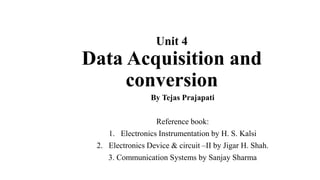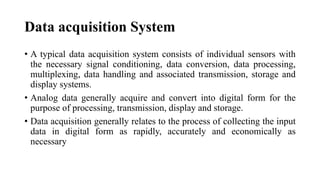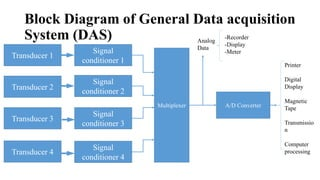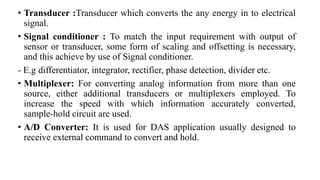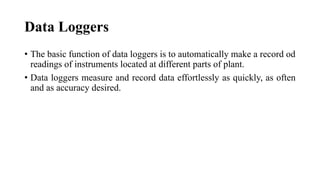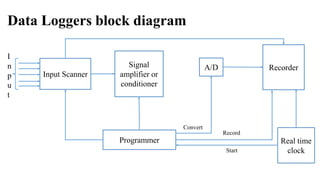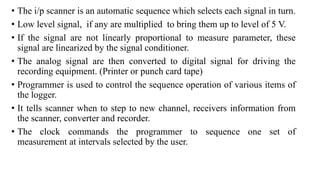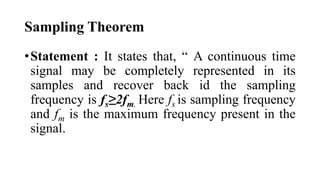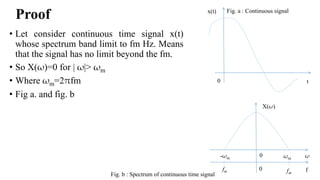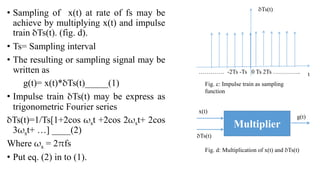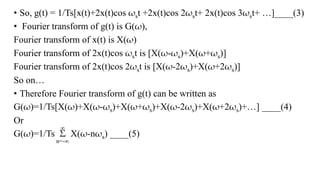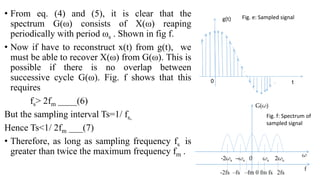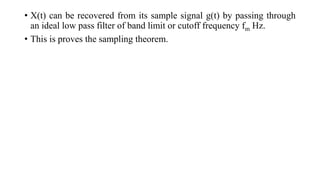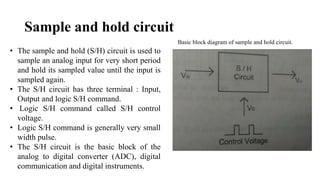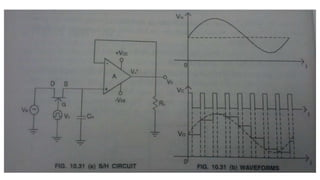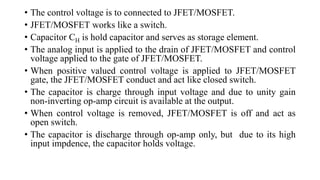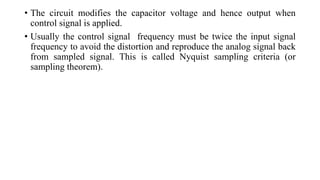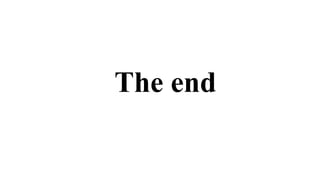1 de 18

### Data acquisition and conversion

• 1. Unit 4 Data Acquisition and conversion By Tejas Prajapati Reference book: 1. Electronics Instrumentation by H. S. Kalsi 2. Electronics Device & circuit –II by Jigar H. Shah. 3. Communication Systems by Sanjay Sharma
• 2. Data acquisition System • A typical data acquisition system consists of individual sensors with the necessary signal conditioning, data conversion, data processing, multiplexing, data handling and associated transmission, storage and display systems. • Analog data generally acquire and convert into digital form for the purpose of processing, transmission, display and storage. • Data acquisition generally relates to the process of collecting the input data in digital form as rapidly, accurately and economically as necessary
• 3. Block Diagram of General Data acquisition System (DAS) Transducer 1 Transducer 2 Transducer 3 Transducer 4 Signal conditioner 1 Signal conditioner 2 Signal conditioner 3 Signal conditioner 4 Multiplexer A/D Converter Printer Digital Display Magnetic Tape Transmissio n Computer processing Analog Data -Recorder -Display -Meter
• 4. • Transducer :Transducer which converts the any energy in to electrical signal. • Signal conditioner : To match the input requirement with output of sensor or transducer, some form of scaling and offsetting is necessary, and this achieve by use of Signal conditioner. - E.g differentiator, integrator, rectifier, phase detection, divider etc. • Multiplexer: For converting analog information from more than one source, either additional transducers or multiplexers employed. To increase the speed with which information accurately converted, sample-hold circuit are used. • A/D Converter: It is used for DAS application usually designed to receive external command to convert and hold.
• 5. Data Loggers • The basic function of data loggers is to automatically make a record od readings of instruments located at different parts of plant. • Data loggers measure and record data effortlessly as quickly, as often and as accuracy desired.
• 6. Data Loggers block diagram Input Scanner Signal amplifier or conditioner A/D Recorder Real time clock Programmer I n p u t Convert Record Start
• 7. • The i/p scanner is an automatic sequence which selects each signal in turn. • Low level signal, if any are multiplied to bring them up to level of 5 V. • If the signal are not linearly proportional to measure parameter, these signal are linearized by the signal conditioner. • The analog signal are then converted to digital signal for driving the recording equipment. (Printer or punch card tape) • Programmer is used to control the sequence operation of various items of the logger. • It tells scanner when to step to new channel, receivers information from the scanner, converter and recorder. • The clock commands the programmer to sequence one set of measurement at intervals selected by the user.
• 8. Sampling Theorem •Statement : It states that, “ A continuous time signal may be completely represented in its samples and recover back id the sampling frequency is fs≥2fm. Here fs is sampling frequency and fm is the maximum frequency present in the signal.
• 9. Proof • Let consider continuous time signal x(t) whose spectrum band limit to fm Hz. Means that the signal has no limit beyond the fm. • So X(ω)=0 for | ω|> ωm • Where ωm=2πfm • Fig a. and fig. b x(t) t0 Fig. a : Continuous signal X(ω) ω f 0 0 ωm-ωm fm fm Fig. b : Spectrum of continuous time signal
• 10. • Sampling of x(t) at rate of fs may be achieve by multiplying x(t) and impulse train δTs(t). (fig. d). • Ts= Sampling interval • The resulting or sampling signal may be written as g(t)= x(t)*δTs(t)_____(1) • Impulse train δTs(t) may be express as trigonometric Fourier series δTs(t)=1/Ts[1+2cos ωst +2cos 2ωst+ 2cos 3ωst+ …] ____(2) Where ωs = 2πfs • Put eq. (2) in to (1). δTs(t) t…………. -2Ts -Ts 0 Ts 2Ts ………….. Multiplier x(t) δTs(t) g(t) Fig. c: Impulse train as sampling function Fig. d: Multiplication of x(t) and δTs(t)
• 11. • So, g(t) = 1/Ts[x(t)+2x(t)cos ωst +2x(t)cos 2ωst+ 2x(t)cos 3ωst+ …]____(3) • Fourier transform of g(t) is G(ω), Fourier transform of x(t) is X(ω) Fourier transform of 2x(t)cos ωst is [X(ω-ωs)+X(ω+ωs)] Fourier transform of 2x(t)cos 2ωst is [X(ω-2ωs)+X(ω+2ωs)] So on… • Therefore Fourier transform of g(t) can be written as G(ω)=1/Ts[X(ω)+X(ω-ωs)+X(ω+ωs)+X(ω-2ωs)+X(ω+2ωs)+…] ____(4) Or G(ω)=1/Ts Σ X(ω-nωs) ____(5) n=-∞ ∞
• 12. • From eq. (4) and (5), it is clear that the spectrum G(ω) consists of X(ω) reaping periodically with period ωs . Shown in fig f. • Now if have to reconstruct x(t) from g(t), we must be able to recover X(ω) from G(ω). This is possible if there is no overlap between successive cycle G(ω). Fig. f shows that this requires fs> 2fm ____(6) But the sampling interval Ts=1/ fs, Hence Ts<1/ 2fm ___(7) • Therefore, as long as sampling frequency fs is greater than twice the maximum frequency fm . g(t) t0 Fig. e: Sampled signal ω f G(ω) -2ωs –ωs 0 ωs 2ωs -2fs –fs –fm 0 fm fs 2fs Fig. f: Spectrum of sampled signal
• 13. • X(t) can be recovered from its sample signal g(t) by passing through an ideal low pass filter of band limit or cutoff frequency fm Hz. • This is proves the sampling theorem.
• 14. Sample and hold circuit Basic block diagram of sample and hold circuit. • The sample and hold (S/H) circuit is used to sample an analog input for very short period and hold its sampled value until the input is sampled again. • The S/H circuit has three terminal : Input, Output and logic S/H command. • Logic S/H command called S/H control voltage. • Logic S/H command is generally very small width pulse. • The S/H circuit is the basic block of the analog to digital converter (ADC), digital communication and digital instruments.
• 16. • The control voltage is to connected to JFET/MOSFET. • JFET/MOSFET works like a switch. • Capacitor CH is hold capacitor and serves as storage element. • The analog input is applied to the drain of JFET/MOSFET and control voltage applied to the gate of JFET/MOSFET. • When positive valued control voltage is applied to JFET/MOSFET gate, the JFET/MOSFET conduct and act like closed switch. • The capacitor is charge through input voltage and due to unity gain non-inverting op-amp circuit is available at the output. • When control voltage is removed, JFET/MOSFET is off and act as open switch. • The capacitor is discharge through op-amp only, but due to its high input impdence, the capacitor holds voltage.
• 17. • The circuit modifies the capacitor voltage and hence output when control signal is applied. • Usually the control signal frequency must be twice the input signal frequency to avoid the distortion and reproduce the analog signal back from sampled signal. This is called Nyquist sampling criteria (or sampling theorem).